# Non-local boundary value problems for impulsive fractional integro-differential equations in Banach spaces

## Abstract

In this study, we establish some conditions for existence and uniqueness of the solutions to semilinear fractional impulsive integro-differential evolution equations with non-local conditions by using Schauder’s fixed point theorem and the contraction mapping principle.

MSC:26A33, 34A37.

## 1 Introduction

The topic of fractional differential equations has received a great deal of attention from many scientists and researchers during the past decades; see, for instance, . This is mostly due to the fact that fractional calculus provides an efficient and excellent instrument to describe many practical dynamical phenomena which arise in engineering and science such as physics, chemistry, biology, economy, viscoelasticity, electrochemistry, electromagnetic, control, porous media; see . Moreover, many researchers study the existence of solutions for fractional differential equations; see  and the references therein.

In particular, several authors have considered a nonlocal Cauchy problem for abstract evolution differential equations having fractional order. Indeed, the nonlocal Cauchy problem for abstract evolution differential equations was studied by Byszewski [17, 18] initially. Afterwards, many authors  discussed the problem for different kinds of nonlinear differential equations and integrodifferential equations including functional differential equations in Banach spaces. Balachandran et al. [22, 23] established the existence of solutions of quasilinear integrodifferential equations with nonlocal conditions. N’Guérékata  and Balachandran and Park  researched the existence of solutions of fractional abstract differential equations with a nonlocal initial condition. Ahmad  obtained some existence results for boundary value problems of fractional semilinear evolution equations. Recently, Balachandran and Trujillo  have investigated the nonlocal Cauchy problem for nonlinear fractional integrodifferential equations in Banach spaces.

On the other hand, the theory of impulsive differential equations for integer order has emerged in mathematical modeling of phenomena and practical situations in both physical and social sciences in recent years. One can see a significant development in impulsive theory. We refer the readers to  for the general theory and applications of impulsive differential equations. Besides, some researchers (see  and the references therein) have addressed the theory of boundary value problems for impulsive fractional differential equations.

However, only a few studies were concerned with the Cauchy problem for impulsive evolution differential equations of fractional order; see . Further, in , Balachandran et al. studied the existence of solutions for fractional impulsive integrodifferential equations of the following type: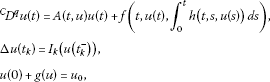where $0\le t\le T$ and $0, by using the contraction mapping principle.

Motivated by the aforementioned works, in this paper, we deal with the existence and uniqueness of solutions for a boundary value problem (BVP), for the following impulsive fractional semilinear integro-differential equation with nonlocal conditions:

$\left\{\begin{array}{c}{}^{C}D^{q}u\left(t\right)=A\left(t\right)u\left(t\right)+f\left(t,u\left(t\right),{\int }_{0}^{t}k\left(t,s,u\left(s\right)\right)\phantom{\rule{0.2em}{0ex}}ds\right),\phantom{\rule{1em}{0ex}}t\in J:=\left[0,1\right],t\ne {t}_{k},\hfill \\ \mathrm{\Delta }u\left({t}_{k}\right)={I}_{k}\left(u\left({t}_{k}^{-}\right)\right),\phantom{\rule{2em}{0ex}}\mathrm{\Delta }{u}^{\mathrm{\prime }}\left({t}_{k}\right)={I}_{k}^{\ast }\left(u\left({t}_{k}^{-}\right)\right),\phantom{\rule{1em}{0ex}}k=1,2,\dots ,p,\hfill \\ \alpha u\left(0\right)+\beta {u}^{\mathrm{\prime }}\left(0\right)={g}_{1}\left(u\right),\phantom{\rule{2em}{0ex}}\alpha u\left(1\right)+\beta {u}^{\mathrm{\prime }}\left(1\right)={g}_{2}\left(u\right),\hfill \end{array}$
(1.1)

where $1, ${}^{C}D^{\alpha }$ is the Caputo fractional derivative, $A\left(t\right)$ is a bounded linear operator on a Banach space X, $f\in C\left(J×X×X,X\right)$,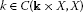, ${I}_{k},{I}_{k}^{\ast }\in C\left(X,X\right)$, ${g}_{1},{g}_{2}:PC\left(J,X\right)\to X$ ($PC\left(J,X\right)$ will be defined in the next section),

$\mathrm{\Delta }u\left({t}_{k}\right)=u\left({t}_{k}^{+}\right)-u\left({t}_{k}^{-}\right)$

with

$u\left({t}_{k}^{+}\right)=\underset{h\to {0}^{+}}{lim}u\left({t}_{k}+h\right),\phantom{\rule{2em}{0ex}}u\left({t}_{k}^{-}\right)=\underset{h\to {0}^{-}}{lim}u\left({t}_{k}+h\right)$

and $\mathrm{\Delta }{u}^{\mathrm{\prime }}\left({t}_{k}\right)$ has a similar meaning for ${u}^{\mathrm{\prime }}\left(t\right)$, $0={t}_{0}<{t}_{1}<{t}_{2}<\cdots <{t}_{p}<{t}_{p+1}=1$, and $\alpha ,\beta \ge 0$. Here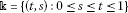. For brevity, let us take $Ku\left(t\right)={\int }_{0}^{t}k\left(t,s,u\left(s\right)\right)\phantom{\rule{0.2em}{0ex}}ds$.

Meanwhile, nonlinear functions f of this type with the integral term k occur in mathematical problems that are concerned with the heat flow in materials having memory and viscoelastic problems; see . Also, as indicated in [40, 41], nonlocal conditions can be more useful than standard conditions to describe physical phenomena. For example, in , the author described the diffusion phenomenon of a small amount of gas in a transparent tube by using the formula

$g\left(u\right)=\sum _{i=1}^{m}{\eta }_{i}u\left({\xi }_{i}\right),$

where ${\eta }_{i}$, $i=1,2,\dots ,m$ are given constants and $0<{\xi }_{1}<{\xi }_{2}<\cdots <{\xi }_{m}.

Note that in this work, to the best of our knowledge, it is the first time that a general boundary value problem for impulsive semilinear evolution integrodifferential equations of fractional order $1 with nonlocal conditions has been considered.

The rest of this paper is organized as follows. In Section 2, we present some notations and preliminary results about fractional calculus and differential equations to be used in the following sections. In Section 3, we discuss some existence and uniqueness results for solutions of BVP (1.1). Namely, the first result is based on Schauder’s fixed point theorem and the second one is based on Banach’s fixed point theorem. Finally, we shall give an illustrative example for our results.

## 2 Preliminaries

In order to model the real world application, the fractional differential equations are considered by using the fractional derivatives. There are many different starting points for the discussion of classical fractional calculus; see, for example, . One can begin with a generalization of repeated integration. If $f\left(t\right)$ is absolutely integrable on $\left[0,b\right)$, it can be found [42, 43]

$\begin{array}{rcl}{\int }_{0}^{t}\phantom{\rule{0.2em}{0ex}}d{t}_{n}{\int }_{0}^{{t}_{n}}\phantom{\rule{0.2em}{0ex}}d{t}_{n-1}\cdots {\int }_{0}^{{t}_{3}}\phantom{\rule{0.2em}{0ex}}d{t}_{2}{\int }_{0}^{t2}f\left({t}_{1}\right)\phantom{\rule{0.2em}{0ex}}d{t}_{1}& =& \frac{1}{\left(n+1\right)!}{\int }_{0}^{t}{\left(t-{t}_{1}\right)}^{n-1}f\left({t}_{1}\right)\phantom{\rule{0.2em}{0ex}}d{t}_{1}\\ =& \frac{1}{\left(n+1\right)!}{t}^{n-1}\ast f\left(t\right),\end{array}$

where $n=1,2,\dots$ and $0\le t\le b$. On writing $\mathrm{\Gamma }\left(n\right)=\left(n-1\right)!$, an immediate generalization in the form of the operation ${I}^{\alpha }$ defined for $\alpha >0$ is

$\begin{array}{rcl}\left({I}^{\alpha }f\right)\left(t\right)& =& \frac{1}{\mathrm{\Gamma }\left(\alpha \right)}{\int }_{0}^{t}{\left(t-{t}_{1}\right)}^{\alpha -1}f\left({t}_{1}\right)\phantom{\rule{0.2em}{0ex}}d{t}_{1}\\ =& \frac{1}{\mathrm{\Gamma }\left(\alpha \right)}{t}^{\alpha -1}\ast f\left(t\right),\phantom{\rule{1em}{0ex}}0\le t
(2.1)

where $\mathrm{\Gamma }\left(\alpha \right)$ is the gamma function and ${t}^{\alpha -1}\ast f\left(t\right)={\int }_{0}^{t}f{\left(t-{t}_{1}\right)}^{\alpha -1}\left({t}_{1}\right)\phantom{\rule{0.2em}{0ex}}d{t}_{1}$ is called the convolution product of ${t}^{\alpha -1}$ and $f\left(t\right)$. Now Eq. (2.1) is known as a fractional integral of order α for the function $f\left(t\right)$.

Next, we give some basic definitions and properties of fractional calculus theory used in this paper; see [1, 4, 28, 31, 32].

Let ${J}_{0}=\left[0,{t}_{1}\right]$, ${J}_{1}=\left({t}_{1},{t}_{2}\right]$, …, ${J}_{k-1}=\left({t}_{k-1},{t}_{k}\right]$, ${J}_{k}=\left({t}_{k},{t}_{k+1}\right]$, and ${J}^{\mathrm{\prime }}:=\left[0,T\right]\mathrm{\setminus }\left\{{t}_{1},{t}_{2},\dots ,{t}_{p}\right\}$, then we define the set of functions as follows:

and

which is a Banach space with the norm

Now, $B\left(X\right)$ denotes the Banach space of bounded linear operators from X into X with the norm ${\parallel A\parallel }_{B\left(X\right)}=sup\left\{\parallel A\left(u\right)\parallel :\parallel u\parallel =1\right\}$.

Definition 1 [1, 4]

The fractional (arbitrary) order integral of the function $h\in {L}^{1}\left(J,{R}_{+}\right)$ of order $q\in {R}_{+}$ is defined by

${I}_{{0}^{+}}^{q}h\left(t\right)=\frac{1}{\mathrm{\Gamma }\left(q\right)}{\int }_{0}^{t}{\left(t-s\right)}^{q-1}h\left(s\right)\phantom{\rule{0.2em}{0ex}}ds,$

where $\mathrm{\Gamma }\left(\cdot \right)$ is the Euler gamma function.

Definition 2 [1, 4]

For a function h given on the interval J, the Caputo-type fractional derivative of order $q>0$ is defined by

${}^{C}D_{{0}^{+}}^{q}h\left(t\right)=\frac{1}{\mathrm{\Gamma }\left(n-q\right)}{\int }_{0}^{t}{\left(t-s\right)}^{n-q-1}{h}^{\left(n\right)}\left(s\right)\phantom{\rule{0.2em}{0ex}}ds,\phantom{\rule{1em}{0ex}}n=\left[q\right]+1,$

where the function $h\left(t\right)$ has absolutely continuous derivatives up to order $\left(n-1\right)$.

Lemma 1 

Let $q>0$, then the differential equation

${}^{C}D^{q}h\left(t\right)=0$

has the following solution:

$h\left(t\right)={c}_{0}+{c}_{1}t+{c}_{2}{t}^{2}+\cdots +{c}_{n-1}{t}^{n-1},\phantom{\rule{1em}{0ex}}{c}_{i}\in R,i=0,1,2,\dots ,n-1,n=\left[q\right]+1.$

Lemma 2 

Let $q>0$, then

${I}^{qC}{D}^{q}h\left(t\right)=h\left(t\right)+{c}_{0}+{c}_{1}t+{c}_{2}{t}^{2}+\cdots +{c}_{n-1}{t}^{n-1}$

for some ${c}_{i}\in R$, $i=0,1,2,\dots ,n-1$, $n=\left[q\right]+1$.

Now, by using the Kronecker convolution product, see , the fractional integral becomes

$\left({I}^{\alpha }f\right)\left(x\right)=\frac{1}{\mathrm{\Gamma }\left(\alpha \right)}{x}^{\alpha -1}\ast f\left(x\right)\simeq {\xi }^{T}\frac{1}{\mathrm{\Gamma }\left(\alpha \right)}\left\{{x}^{\alpha -1}\ast {\varphi }_{m}\left(x\right)\right\}.$
(2.2)

Thus, if ${x}^{\alpha -1}\ast {\varphi }_{m}\left(x\right)$ can be integrated, then expanded in block pulse functions, the fractional integral is solved via the block pulse functions operational matrix as follows:

$\frac{1}{\mathrm{\Gamma }\left(\alpha \right)}{\int }_{0}^{t}{\left(t-{t}_{1}\right)}^{\alpha -1}{\varphi }_{m}\left({t}_{1}\right)\phantom{\rule{0.2em}{0ex}}d{t}_{1}\simeq {F}_{\alpha }{\varphi }_{m}\left(t\right),$

where

${\psi }_{m}\left(t\right)=\left\{\begin{array}{cc}1\hfill & \left(\frac{m-1}{i}\right)b\le t<\left(\frac{m}{i}\right)b,\hfill \\ 0\hfill & \text{elsewhere}\hfill \end{array}$

for $m=1,2,\dots ,i$ and

${F}_{\alpha }={\left(\frac{b}{m}\right)}^{\alpha }\frac{1}{\mathrm{\Gamma }\left(\alpha +2\right)}\left[\begin{array}{ccccc}1& {\xi }_{2}& {\xi }_{3}& \cdots & {\xi }_{m}\\ 0& 1& {\xi }_{2}& \cdots & {\xi }_{m-1}\\ 0& 0& 1& \cdots & {\xi }_{m-2}\\ 0& 0& 0& \ddots & ⋮\\ 0& 0& 0& 0& 1\end{array}\right];$

see .

Now, we need the following lemma for our study.

Lemma 3 Let $1 and $h:J\to X$ be continuous. A function $u\left(t\right)$ is a solution of the fractional integral equation

$u\left(t\right)=\left\{\begin{array}{c}{\int }_{0}^{t}\frac{{\left(t-s\right)}^{q-1}}{\mathrm{\Gamma }\left(q\right)}h\left(s\right)\phantom{\rule{0.2em}{0ex}}ds+\left(\frac{\beta }{\alpha }-t\right)\left[{\int }_{{t}_{k}}^{1}\frac{{\left(1-s\right)}^{q-1}}{\mathrm{\Gamma }\left(q\right)}h\left(s\right)\phantom{\rule{0.2em}{0ex}}ds+\frac{\beta }{\alpha }{\int }_{{t}_{k}}^{1}\frac{{\left(1-s\right)}^{q-2}}{\mathrm{\Gamma }\left(q-1\right)}h\left(s\right)\phantom{\rule{0.2em}{0ex}}ds\hfill \\ \phantom{\rule{1em}{0ex}}+{\sum }_{i=1}^{k}\left({\int }_{{t}_{i-1}}^{{t}_{i}}\frac{{\left({t}_{i}-s\right)}^{q-1}}{\mathrm{\Gamma }\left(q\right)}h\left(s\right)\phantom{\rule{0.2em}{0ex}}ds+{I}_{i}\left(u\left({t}_{i}^{-}\right)\right)\right)\hfill \\ \phantom{\rule{1em}{0ex}}+{\sum }_{i=1}^{k}\left(\frac{\beta }{\alpha }+1-{t}_{k}\right)\left({\int }_{{t}_{i-1}}^{{t}_{i}}\frac{{\left({t}_{1}-s\right)}^{q-2}}{\mathrm{\Gamma }\left(q-1\right)}h\left(s\right)\phantom{\rule{0.2em}{0ex}}ds+{I}_{i}^{\ast }\left(u\left({t}_{i}^{-}\right)\right)\right)\hfill \\ \phantom{\rule{1em}{0ex}}+\frac{1}{\alpha }\left({g}_{1}\left(u\right)-{g}_{2}\left(u\right)\right)\right]+\frac{{g}_{1}\left(u\right)}{\alpha },\hfill & t\in {J}_{0},\hfill \\ {\int }_{{t}_{k}}^{t}\frac{{\left(t-s\right)}^{q-1}}{\mathrm{\Gamma }\left(q\right)}h\left(s\right)\phantom{\rule{0.2em}{0ex}}ds+{\sum }_{i=1}^{k}\left({\int }_{{t}_{i-1}}^{{t}_{i}}\frac{{\left({t}_{i}-s\right)}^{q-1}}{\mathrm{\Gamma }\left(q\right)}h\left(s\right)\phantom{\rule{0.2em}{0ex}}ds+{I}_{i}\left(u\left({t}_{i}^{-}\right)\right)\right)\hfill \\ \phantom{\rule{1em}{0ex}}+{\sum }_{i=1}^{k}\left(t-{t}_{i}\right){\int }_{{t}_{i-1}}^{{t}_{i}}\left(\frac{{\left({t}_{i}-s\right)}^{q-2}}{\mathrm{\Gamma }\left(q-1\right)}h\left(s\right)\phantom{\rule{0.2em}{0ex}}ds+{I}_{i}^{\ast }\left(u\left({t}_{i}^{-}\right)\right)\right)\hfill \\ \phantom{\rule{1em}{0ex}}+\left(\frac{\beta }{\alpha }-t\right)\left[{\int }_{{t}_{k}}^{1}\frac{{\left(1-s\right)}^{q-1}}{\mathrm{\Gamma }\left(q\right)}h\left(s\right)\phantom{\rule{0.2em}{0ex}}ds+\frac{\beta }{\alpha }{\int }_{{t}_{k}}^{1}\frac{{\left(1-s\right)}^{q-2}}{\mathrm{\Gamma }\left(q-1\right)}h\left(s\right)\phantom{\rule{0.2em}{0ex}}ds\hfill \\ \phantom{\rule{1em}{0ex}}+{\sum }_{i=1}^{k}\left({\int }_{{t}_{i-1}}^{{t}_{i}}\frac{{\left({t}_{i}-s\right)}^{q-1}}{\mathrm{\Gamma }\left(q\right)}h\left(s\right)\phantom{\rule{0.2em}{0ex}}ds+{I}_{i}\left(u\left({t}_{i}^{-}\right)\right)\right)\hfill \\ \phantom{\rule{1em}{0ex}}+{\sum }_{i=1}^{k}\left(\frac{\beta }{\alpha }+1-{t}_{k}\right)\left({\int }_{{t}_{i-1}}^{{t}_{i}}\frac{{\left({t}_{1}-s\right)}^{q-2}}{\mathrm{\Gamma }\left(q-1\right)}h\left(s\right)\phantom{\rule{0.2em}{0ex}}ds+{I}_{i}^{\ast }\left(u\left({t}_{i}^{-}\right)\right)\right)\hfill \\ \phantom{\rule{1em}{0ex}}+\frac{1}{\alpha }\left({g}_{1}\left(u\right)-{g}_{2}\left(u\right)\right)\right]+\frac{{g}_{1}\left(u\right)}{\alpha },\hfill & t\in {J}_{k}\hfill \end{array}$
(2.3)

if and only if $u\left(t\right)$ is a solution of the fractional BVP

$\begin{array}{r}{}^{C}D^{q}u\left(t\right)=h\left(t\right),\phantom{\rule{1em}{0ex}}t\in {J}^{\mathrm{\prime }}\\ \mathrm{\Delta }u\left({t}_{k}\right)={I}_{k}\left(u\left({t}_{k}^{-}\right)\right),\phantom{\rule{2em}{0ex}}\mathrm{\Delta }{u}^{\mathrm{\prime }}\left({t}_{k}\right)={I}_{k}^{\ast }\left(u\left({t}_{k}^{-}\right)\right),\\ \alpha u\left(0\right)+\beta {u}^{\mathrm{\prime }}\left(0\right)={g}_{1}\left(u\right),\phantom{\rule{2em}{0ex}}\alpha u\left(1\right)+\beta {u}^{\mathrm{\prime }}\left(1\right)={g}_{2}\left(u\right),\end{array}$
(2.4)

where $k=1,2,\dots ,p$.

Proof Let u be the solution of (2.4). If $t\in {J}_{0}$, then Lemma 2 implies that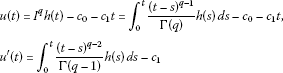for some ${c}_{0},{c}_{1}\in R$.

Applying the boundary condition $\alpha u\left(0\right)+\beta {u}^{\mathrm{\prime }}\left(0\right)={g}_{1}\left(u\right)$ for $t\in {J}_{0}$, we find that

$u\left(t\right)={\int }_{0}^{t}\frac{{\left(t-s\right)}^{q-1}}{\mathrm{\Gamma }\left(q\right)}h\left(s\right)\phantom{\rule{0.2em}{0ex}}ds+{c}_{1}\left(\frac{\beta }{\alpha }-t\right)+\frac{{g}_{1}\left(u\right)}{\alpha }.$
(2.5)

If $t\in {J}_{1}$, then Lemma 2 implies that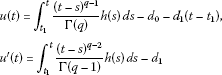for some ${d}_{0},{d}_{1}\in R$. Thus, we have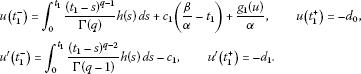In the view of

$\mathrm{\Delta }u\left({t}_{1}\right)=u\left({t}_{1}^{+}\right)-u\left({t}_{1}^{-}\right)={I}_{1}\left(u\left({t}_{1}^{-}\right)\right)\phantom{\rule{1em}{0ex}}\text{and}\phantom{\rule{1em}{0ex}}\mathrm{\Delta }{u}^{\mathrm{\prime }}\left({t}_{1}\right)={u}^{\mathrm{\prime }}\left({t}_{1}^{+}\right)-{u}^{\mathrm{\prime }}\left({t}_{1}^{-}\right)={I}_{1}^{\ast }\left(u\left({t}_{1}^{-}\right)\right),$

we have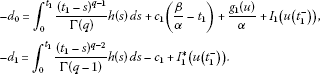Hence,

By repeating the process, for $t\in {J}_{k}$, we have

$\begin{array}{rcl}u\left(t\right)& =& {\int }_{{t}_{k}}^{t}\frac{{\left(t-s\right)}^{q-1}}{\mathrm{\Gamma }\left(q\right)}h\left(s\right)\phantom{\rule{0.2em}{0ex}}ds+\sum _{i=1}^{k}\left[{\int }_{{t}_{i-1}}^{{t}_{i}}\frac{{\left({t}_{i}-s\right)}^{q-1}}{\mathrm{\Gamma }\left(q\right)}h\left(s\right)\phantom{\rule{0.2em}{0ex}}ds+{I}_{i}\left(u\left({t}_{i}^{-}\right)\right)\right]\\ +\sum _{i=1}^{k}\left(t-{t}_{i}\right)\left[{\int }_{{t}_{i-1}}^{{t}_{i}}\frac{{\left({t}_{i}-s\right)}^{q-2}}{\mathrm{\Gamma }\left(q-1\right)}h\left(s\right)\phantom{\rule{0.2em}{0ex}}ds+{I}_{i}^{\ast }\left(u\left({t}_{i}^{-}\right)\right)\right]\\ +{c}_{1}\left(\frac{\beta }{\alpha }-t\right)+\frac{{g}_{1}\left(u\right)}{\alpha }.\end{array}$
(2.6)

Now, applying the boundary condition

$\alpha u\left(1\right)+\beta {u}^{\mathrm{\prime }}\left(1\right)={g}_{2}\left(u\right),$

we find that

$\begin{array}{rcl}{c}_{1}& =& {\int }_{{t}_{k}}^{1}\frac{{\left(1-s\right)}^{q-1}}{\mathrm{\Gamma }\left(q\right)}h\left(s\right)\phantom{\rule{0.2em}{0ex}}ds+\frac{\beta }{\alpha }{\int }_{{t}_{k}}^{1}\frac{{\left(1-s\right)}^{q-2}}{\mathrm{\Gamma }\left(q-1\right)}h\left(s\right)\phantom{\rule{0.2em}{0ex}}ds\\ +\sum _{i=1}^{k}\left[{\int }_{{t}_{i-1}}^{{t}_{i}}\frac{{\left({t}_{i}-s\right)}^{q-1}}{\mathrm{\Gamma }\left(q\right)}h\left(s\right)\phantom{\rule{0.2em}{0ex}}ds+{I}_{i}\left(u\left({t}_{i}^{-}\right)\right)\right]\\ +\sum _{i=1}^{k}\left(\frac{\beta }{\alpha }+1-{t}_{k}\right)\left[{\int }_{{t}_{i-1}}^{{t}_{i}}\frac{{\left({t}_{i}-s\right)}^{q-2}}{\mathrm{\Gamma }\left(q-1\right)}h\left(s\right)\phantom{\rule{0.2em}{0ex}}ds+{I}_{i}^{\ast }\left(u\left({t}_{i}^{-}\right)\right)\right]\\ +\frac{1}{\alpha }\left[{g}_{1}\left(u\right)-{g}_{2}\left(u\right)\right].\end{array}$

Substituting the value of ${c}_{1}$ in (2.5) and (2.6), we obtain Eq. 2.3.

Conversely, if we assume that u satisfies the impulsive fractional integral equation (2.3), then by direct computation, we can easily see that the solution given by (2.3) satisfies (2.4). Thus, the proof of Lemma 3 is complete. □

## 3 Main results

Definition 3 A function $u\in P{C}^{1}\left(J,X\right)$ with its q-derivative existing on ${J}^{\mathrm{\prime }}$ is said to be a solution of (1.1) if u satisfies the equation

${}^{C}D^{q}u\left(t\right)=A\left(t\right)u\left(t\right)+f\left(t,u\left(t\right),Ku\left(t\right)\right)$

on ${J}^{\mathrm{\prime }}$ and satisfies the conditions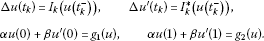Now, we define the operator $T:P{C}^{1}\left(J,X\right)\to P{C}^{1}\left(J,X\right)$ by

$Tu\left(t\right)=\left\{\begin{array}{c}{\int }_{{t}_{k}}^{t}\frac{{\left(t-s\right)}^{q-1}}{\mathrm{\Gamma }\left(q\right)}\left(A\left(s\right)u\left(s\right)+f\left(s,u\left(s\right),Ku\left(s\right)\right)\right)\phantom{\rule{0.2em}{0ex}}ds\hfill \\ \phantom{\rule{1em}{0ex}}+{\sum }_{i=1}^{k}\left({\int }_{{t}_{i-1}}^{{t}_{i}}\frac{{\left({t}_{i}-s\right)}^{q-1}}{\mathrm{\Gamma }\left(q\right)}\left(A\left(s\right)u\left(s\right)+f\left(s,u\left(s\right),Ku\left(s\right)\right)\right)\phantom{\rule{0.2em}{0ex}}ds+{I}_{i}\left(u\left({t}_{i}^{-}\right)\right)\right)\hfill \\ \phantom{\rule{1em}{0ex}}+{\sum }_{i=1}^{k}\left(t-{t}_{i}\right)\hfill \\ \phantom{\rule{1em}{0ex}}×\left({\int }_{{t}_{i-1}}^{{t}_{i}}\frac{{\left({t}_{i}-s\right)}^{q-2}}{\mathrm{\Gamma }\left(q-1\right)}\left(A\left(s\right)u\left(s\right)+f\left(s,u\left(s\right),Ku\left(s\right)\right)\right)\phantom{\rule{0.2em}{0ex}}ds+{I}_{i}^{\ast }\left(u\left({t}_{i}^{-}\right)\right)\right)\hfill \\ \phantom{\rule{1em}{0ex}}+\left(\frac{\beta }{\alpha }-t\right)\left[{\int }_{{t}_{k}}^{1}\frac{{\left(1-s\right)}^{q-1}}{\mathrm{\Gamma }\left(q\right)}\left(A\left(s\right)u\left(s\right)+f\left(s,u\left(s\right),Ku\left(s\right)\right)\right)\phantom{\rule{0.2em}{0ex}}ds\hfill \\ \phantom{\rule{1em}{0ex}}+\frac{\beta }{\alpha }{\int }_{{t}_{k}}^{1}\frac{{\left(1-s\right)}^{q-2}}{\mathrm{\Gamma }\left(q-1\right)}\left(A\left(s\right)u\left(s\right)+f\left(s,u\left(s\right),Ku\left(s\right)\right)\right)\phantom{\rule{0.2em}{0ex}}ds\hfill \\ \phantom{\rule{1em}{0ex}}+{\sum }_{i=1}^{k}\left({\int }_{{t}_{i-1}}^{{t}_{i}}\frac{{\left({t}_{i}-s\right)}^{q-1}}{\mathrm{\Gamma }\left(q\right)}\left(A\left(s\right)u\left(s\right)+f\left(s,u\left(s\right),Ku\left(s\right)\right)\right)\phantom{\rule{0.2em}{0ex}}ds+{I}_{i}\left(u\left({t}_{i}^{-}\right)\right)\right)\hfill \\ \phantom{\rule{1em}{0ex}}+{\sum }_{i=1}^{k}\left(\frac{\beta }{\alpha }+1-{t}_{k}\right)\hfill \\ \phantom{\rule{1em}{0ex}}×\left({\int }_{{t}_{i-1}}^{{t}_{i}}\frac{{\left({t}_{1}-s\right)}^{q-2}}{\mathrm{\Gamma }\left(q-1\right)}\left(A\left(s\right)u\left(s\right)+f\left(s,u\left(s\right),Ku\left(s\right)\right)\right)\phantom{\rule{0.2em}{0ex}}ds+{I}_{i}^{\ast }\left(u\left({t}_{i}^{-}\right)\right)\right)\hfill \\ \phantom{\rule{1em}{0ex}}+\frac{1}{\alpha }\left({g}_{1}\left(u\right)-{g}_{2}\left(u\right)\right)\right]+\frac{{g}_{1}\left(u\right)}{\alpha },\phantom{\rule{1em}{0ex}}t\in {J}_{k}.\hfill \end{array}$
(3.1)

Clearly, the fixed points of the operator T are the solutions of problem (1.1). To begin with, we need the following assumptions to prove the existence and uniqueness of a solution of the integral equation (2.3) which satisfies BVP (1.1):

(A1) $A:X\to X$ is a continuous bounded linear operator and there exists a constant ${A}_{1}>0$ such that ${\parallel A\left(u\right)\parallel }_{B\left(X\right)}\le {A}_{1}$ for all $u\in X$;

(A2) The function $f:J×X×X\to X$ is continuous and there exists a constant ${M}_{1}>0$ such that ${M}_{1}={max}_{s\in J}\left\{f\left(s,u\left(s\right),Ku\left(s\right)\right),u\in X\right\}$;

(A3) ${I}_{k},{I}_{k}^{\ast }:X\to X$ are continuous and there exist constants ${M}_{2}>0$ and ${M}_{3}>0$ such that $\parallel {I}_{k}\left(u\right)\parallel \le {M}_{2},\parallel {I}_{k}^{\ast }\left(u\right)\parallel \le {M}_{3}$ for each $u\in X$ and $k=1,2,\dots ,p$;

(A4) There exist constants${G}_{i}>0$ and ${g}_{i}:P{C}^{1}\left(J,X\right)\to X$ are continuous functions such that $\parallel {g}_{i}\left(u\right)\parallel \le {G}_{i}$, $i=1,2$;

(A5) There exists a constant ${L}_{1}>0$ such that

$\parallel f\left(t,{u}_{1},{v}_{1}\right)-f\left(t,{u}_{2},{v}_{2}\right)\parallel \le {L}_{1}\left(\parallel {u}_{1}-{u}_{2}\parallel +\parallel {v}_{1}-{v}_{2}\parallel \right),$

$\mathrm{\forall }t\in J$, and ${u}_{1},{u}_{2},{v}_{1},{v}_{2}\in X$;

(A6)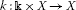is continuous and there exists a constant ${L}_{2}>0$ such that

$\parallel k\left(t,s,u\right)-k\left(t,s,v\right)\parallel \le {L}_{2}\parallel u-v\parallel$

for all $u,v\in X$;

(A7) There exist constants ${L}_{3}>0$, ${L}_{4}>0$ such that $\parallel {I}_{k}\left(u\right)-{I}_{k}\left(v\right)\parallel \le {L}_{3}\parallel u-v\parallel$, $\parallel {I}_{k}^{\ast }\left(u\right)-{I}_{k}^{\ast }\left(v\right)\parallel \le {L}_{4}\parallel u-v\parallel$ for each $u,v\in X$ and $k=1,2,\dots ,p$;

(A8) There exist constants ${b}_{i}>0$ such that $\parallel {g}_{i}\left(u\right)-{g}_{i}\left(v\right)\parallel \le {b}_{i}\parallel u-v\parallel$, $i=1,2$.

The following are the main results of this paper. Our first result relies on Schauder’s fixed point theorem which gives an existence result for solutions of BVP (1.1).

Theorem 1 Assume that the assumptions (A1)-(A4) hold. Then BVP (1.1) has at least one solution on J.

Proof In order to show the existence of a solution of BVP (1.1), we need to transform BVP (1.1) to a fixed point problem by using the operator T in (3.1). Now, we shall use Schauder’s fixed point theorem to prove T has a fixed point which is then a solution of BVP (1.1). First, let us define ${B}_{r}=\left\{u\in P{C}^{1}\left(J\right):\parallel u\parallel \le r\right\}$ for any $r>0$. Then it is clear that the set ${B}_{r}$ is a closed, bounded and convex. The proof will be given in several steps.

Step 1: T is continuous.

Let $\left\{{u}_{n}\right\}$ be a sequence such that ${u}_{n}\to u$ in $PC\left(J\right)$. Then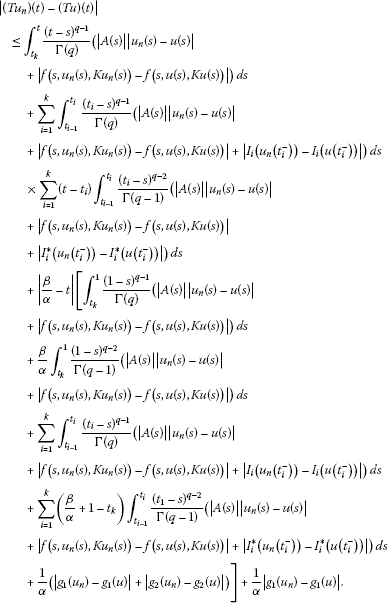Since A is a continuous operator and f, g, I, ${I}^{\ast }$ are continuous functions, we have $\parallel T{u}_{n}-Tu\parallel \to 0$ as $n\to \mathrm{\infty }$.

Step 2: T maps bounded sets into bounded sets.

Now, it is enough to show that there exists a positive constant l such that $\parallel Tu\parallel \le l$ for each $u\in {B}_{r}$. Then we have, for each $t\in J$,

$\begin{array}{rcl}|\left(Tu\right)\left(t\right)|& \le & {\int }_{{t}_{k}}^{t}\frac{{\left(t-s\right)}^{q-1}}{\mathrm{\Gamma }\left(q\right)}\left(|A\left(s\right)||u\left(s\right)|+|f\left(s,u\left(s\right),Ku\left(s\right)\right)|\right)\phantom{\rule{0.2em}{0ex}}ds\\ +\sum _{i=1}^{k}{\int }_{{t}_{i-1}}^{{t}_{i}}\frac{{\left({t}_{i}-s\right)}^{q-1}}{\mathrm{\Gamma }\left(q\right)}\left(|A\left(s\right)||u\left(s\right)|+|f\left(s,u\left(s\right),Ku\left(s\right)\right)|+|{I}_{i}\left(u\left({t}_{i}^{-}\right)\right)|\right)\phantom{\rule{0.2em}{0ex}}ds\\ +\sum _{i=1}^{k}\left(t-{t}_{i}\right){\int }_{{t}_{i-1}}^{{t}_{i}}\frac{{\left({t}_{i}-s\right)}^{q-2}}{\mathrm{\Gamma }\left(q-1\right)}\left(|A\left(s\right)||u\left(s\right)|+|f\left(s,u\left(s\right),Ku\left(s\right)\right)|\\ +|{I}_{i}^{\ast }\left(u\left({t}_{i}^{-}\right)\right)|\right)\phantom{\rule{0.2em}{0ex}}ds\\ +|\frac{\beta }{\alpha }-t|\left[{\int }_{{t}_{k}}^{1}\frac{{\left(1-s\right)}^{q-1}}{\mathrm{\Gamma }\left(q\right)}\left(|A\left(s\right)||u\left(s\right)|+|f\left(s,u\left(s\right),Ku\left(s\right)\right)|\right)\phantom{\rule{0.2em}{0ex}}ds\\ +\frac{\beta }{\alpha }{\int }_{{t}_{k}}^{1}\frac{{\left(1-s\right)}^{q-2}}{\mathrm{\Gamma }\left(q-1\right)}\left(|A\left(s\right)||u\left(s\right)|+|f\left(s,u\left(s\right),Ku\left(s\right)\right)|\right)\phantom{\rule{0.2em}{0ex}}ds\\ +\sum _{i=1}^{k}{\int }_{{t}_{i-1}}^{{t}_{i}}\frac{{\left({t}_{i}-s\right)}^{q-1}}{\mathrm{\Gamma }\left(q\right)}\left(|A\left(s\right)||u\left(s\right)|+|f\left(s,u\left(s\right),Ku\left(s\right)\right)|+|{I}_{i}\left(u\left({t}_{i}^{-}\right)\right)|\right)\phantom{\rule{0.2em}{0ex}}ds\\ +\sum _{i=1}^{k}\left(\frac{\beta }{\alpha }+1-{t}_{k}\right){\int }_{{t}_{i-1}}^{{t}_{i}}\frac{{\left({t}_{1}-s\right)}^{q-2}}{\mathrm{\Gamma }\left(q-1\right)}\left(|A\left(s\right)||u\left(s\right)|+|f\left(s,u\left(s\right),Ku\left(s\right)\right)|\\ +|{I}_{i}^{\ast }\left(u\left({t}_{i}^{-}\right)\right)|\right)\phantom{\rule{0.2em}{0ex}}ds\\ +\frac{1}{\alpha }\left(|{g}_{1}\left(u\right)|+|{g}_{2}\left(u\right)|\right)\right]+\frac{1}{\alpha }|{g}_{1}\left(u\right)|.\end{array}$

Thus,

$\begin{array}{rcl}|\left(Tu\right)\left(t\right)|& \le & \left({A}_{1}r+{M}_{1}\right){\int }_{{t}_{k}}^{t}\frac{{\left(t-s\right)}^{q-1}}{\mathrm{\Gamma }\left(q\right)}\phantom{\rule{0.2em}{0ex}}ds+\left({A}_{1}r+{M}_{1}+{M}_{2}\right)\sum _{i=1}^{k}{\int }_{{t}_{i-1}}^{{t}_{i}}\frac{{\left({t}_{i}-s\right)}^{q-1}}{\mathrm{\Gamma }\left(q\right)}\phantom{\rule{0.2em}{0ex}}ds\\ +\left({A}_{1}r+{M}_{1}+{M}_{3}\right)\sum _{i=1}^{k}|t-{t}_{i}|{\int }_{{t}_{i-1}}^{{t}_{i}}\frac{{\left({t}_{i}-s\right)}^{q-2}}{\mathrm{\Gamma }\left(q-1\right)}\phantom{\rule{0.2em}{0ex}}ds\\ +\left(\frac{\beta }{\alpha }+1\right)\left[\left({A}_{1}r+{M}_{1}\right)\left({\int }_{{t}_{k}}^{1}\frac{{\left(1-s\right)}^{q-1}}{\mathrm{\Gamma }\left(q\right)}\phantom{\rule{0.2em}{0ex}}ds+\frac{\beta }{\alpha }{\int }_{{t}_{k}}^{1}\frac{{\left(1-s\right)}^{q-2}}{\mathrm{\Gamma }\left(q-1\right)}\phantom{\rule{0.2em}{0ex}}ds\right)\\ +\left({A}_{1}r+{M}_{1}+{M}_{2}\right)\sum _{i=1}^{k}{\int }_{{t}_{i-1}}^{{t}_{i}}\frac{{\left({t}_{i}-s\right)}^{q-1}}{\mathrm{\Gamma }\left(q\right)}\phantom{\rule{0.2em}{0ex}}ds\\ +\left({A}_{1}r+{M}_{1}+{M}_{3}\right)\sum _{i=1}^{k}\left(\frac{\beta }{\alpha }+1\right){\int }_{{t}_{i-1}}^{{t}_{i}}\frac{{\left({t}_{1}-s\right)}^{q-2}}{\mathrm{\Gamma }\left(q-1\right)}\phantom{\rule{0.2em}{0ex}}ds\\ +\frac{1}{\alpha }\left({G}_{1}+{G}_{2}\right)\right]+\frac{1}{\alpha }{G}_{1}\\ \le & \frac{1}{\mathrm{\Gamma }\left(q+1\right)}\left(\frac{\beta }{\alpha }+2\right)\left(\left({A}_{1}r+{M}_{1}\right)\left(1+p\right)+p{M}_{2}\right)\\ +\frac{1}{\mathrm{\Gamma }\left(q\right)}\left[p\left({A}_{1}r+{M}_{1}+{M}_{3}\right)\left(1+{\left(\frac{\beta }{\alpha }+1\right)}^{2}\right)+\left({A}_{1}r+{M}_{1}\right)\frac{\beta }{\alpha }\left(\frac{\beta }{\alpha }+1\right)\right]\\ +\frac{1}{\alpha }\left(\frac{\beta }{\alpha }+1\right)\left({G}_{1}+{G}_{2}\right)+{G}_{1}=l.\end{array}$

Then it follows that $\parallel Tu\parallel \le l$.

Step 3: T maps bounded sets into equicontinuous sets.

Let ${B}_{r}$ be a bounded set of $P{C}^{1}\left(J\right)$ as in Step 2, and let $u\in {B}_{r}$. Then, letting ${\tau }_{1},{\tau }_{2}\in {J}_{k}$ with ${\tau }_{1}<{\tau }_{2}$, $0\le k\le p$, we have

$|\left(Tu\right)\left({\tau }_{2}\right)-\left(Tu\right)\left({\tau }_{1}\right)|\le {\int }_{{\tau }_{1}}^{{\tau }_{2}}|{\left(Tu\right)}^{\mathrm{\prime }}\left(s\right)|\phantom{\rule{0.2em}{0ex}}ds\le \stackrel{˜}{l}\left({\tau }_{2}-{\tau }_{1}\right),$

where

Hence, $T\left({B}_{r}\right)$ is equicontinuous on all the subintervals ${J}_{k}$, $k=0,1,2,\dots ,p$. Then we can deduce that $T:P{C}^{1}\left(J,X\right)\to P{C}^{1}\left(J,X\right)$ is completely continuous as a result of the Arzela-Ascoli theorem together with Steps 1 to 3.

As a consequence of Schauder’s fixed point theorem, we conclude that T has a fixed point. That is, BVP (1.1) has at least one solution. The proof is complete. □

Our second result is about the uniqueness of the solution of BVP (1.1). And it depends on Banach’s fixed point theorem.

Theorem 2 Assume that (A1)-(A8) hold with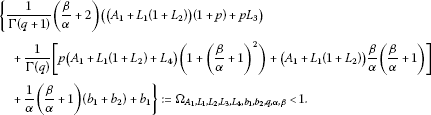(3.2)

Proof First, we show that $T{B}_{r}\subset {B}_{r}$. Indeed, in order to do this, it is adequate to replace l with r in Step 2 in Theorem 1. Thus, T maps ${B}_{r}$ into itself. Now, define the mapping $T:C\left(J,{B}_{r}\right)\to C\left(J,{B}_{r}\right)$. Then, for each $t\in J$, we have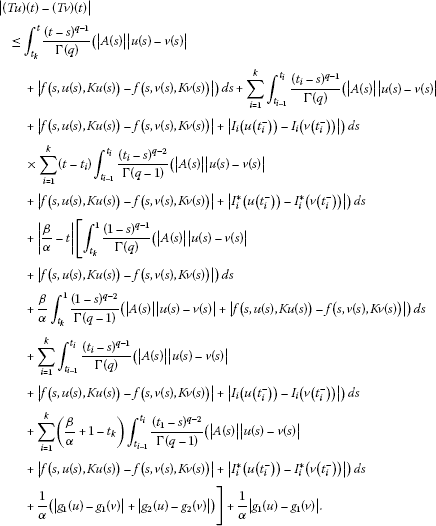Observing the inequality

$\begin{array}{rcl}|f\left(s,u\left(s\right),Ku\left(s\right)\right)-f\left(s,v\left(s\right),Kv\left(s\right)\right)|& \le & {L}_{1}\left(|u\left(s\right)-v\left(s\right)|+|Ku\left(s\right)-Kv\left(s\right)|\right)\\ \le & {L}_{1}\left(1+{L}_{2}\right)|u\left(s\right)-v\left(s\right)|,\end{array}$

we have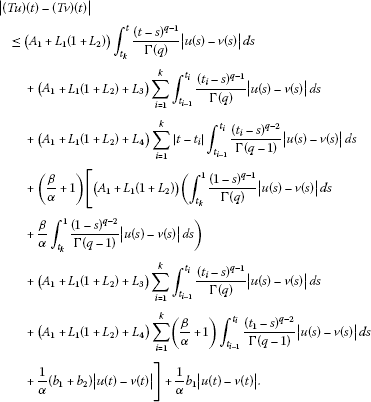Thus,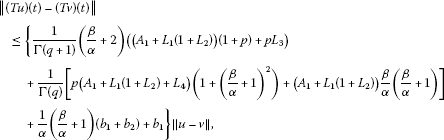which implies that

$\parallel \left(Tu\right)\left(t\right)-\left(Tv\right)\left(t\right)\parallel \le {\mathrm{\Omega }}_{{A}_{1},{L}_{1},{L}_{2},{L}_{3},{L}_{4},{b}_{1},{b}_{2},q,\alpha ,\beta }\parallel u-v\parallel .$

Therefore, by (3.2), the operator T is a contraction. As a consequence of Banach’s fixed point theorem, we deduce that T has a fixed point which is a unique solution of BVP (1.1). □

Example 1 Consider the following boundary value problem for impulsive integrodifferential evolution equation of fractional order:

$\begin{array}{r}{}^{C}D^{\frac{3}{2}}u\left(t\right)=\frac{1}{20}\left({cos}^{2}t\right)u\left(t\right)+\frac{\left(sin7t\right)|u\left(t\right)|}{{\left(t+5\right)}^{4}\left(1+|u\left(t\right)|\right)}\\ \phantom{{}^{C}D^{\frac{3}{2}}u\left(t\right)=}+{\int }_{0}^{t}{e}^{-\frac{1}{25}u\left(s\right)}\phantom{\rule{0.2em}{0ex}}ds,\phantom{\rule{1em}{0ex}}t\in \left[0,1\right],t\ne \frac{1}{2},\\ \mathrm{\Delta }u\left(\frac{1}{2}\right)=\frac{|u\left({\frac{1}{2}}^{-}\right)|}{15+|u\left({\frac{1}{2}}^{-}\right)|},\phantom{\rule{2em}{0ex}}\mathrm{\Delta }{u}^{\mathrm{\prime }}\left(\frac{1}{2}\right)=\frac{|{u}^{\mathrm{\prime }}\left({\frac{1}{2}}^{-}\right)|}{10+|{u}^{\mathrm{\prime }}\left({\frac{1}{2}}^{-}\right)|}\\ 3u\left(0\right)+{u}^{\mathrm{\prime }}\left(0\right)=\sum _{i=1}^{m}{\eta }_{i}u\left({\xi }_{i}\right),\phantom{\rule{2em}{0ex}}3u\left(1\right)+{u}^{\mathrm{\prime }}\left(1\right)=\sum _{j=1}^{m}{\stackrel{˜}{\eta }}_{j}\stackrel{˜}{u}\left({\xi }_{i}\right),\end{array}$
(3.3)

where $0<{\eta }_{1}<{\eta }_{2}<\cdots <1$, $0<{\stackrel{˜}{\eta }}_{1}<{\stackrel{˜}{\eta }}_{2}<\cdots <1$, and ${\eta }_{i}$, ${\stackrel{˜}{\eta }}_{j}$ are given positive constants with ${\sum }_{i=1}^{m}{\eta }_{i}<\frac{2}{15}$ and ${\sum }_{j=1}^{m}{\stackrel{˜}{\eta }}_{j}<\frac{3}{15}$.

Here, $\alpha =3$, $\beta =1$, $q=\frac{3}{2}$, $p=1$. Obviously, ${A}_{1}=\frac{1}{10}$, ${L}_{1}=\frac{1}{125}$, ${L}_{2}=\frac{1}{25}$, ${L}_{3}=\frac{1}{15}$, ${L}_{4}=\frac{1}{10}$, ${b}_{1}=\frac{2}{15}$, ${b}_{2}=\frac{3}{15}$ and by (2.5), it can be found that

${\mathrm{\Omega }}_{{A}_{1},{L}_{1},{L}_{2},{L}_{3},{L}_{4},{b}_{1},{b}_{2},q,\alpha ,\beta }=\frac{1,203,709}{843,750\sqrt{\pi }}+\frac{38}{135}=0.63361<1.$

Therefore, due to the fact that all the assumptions of Theorem 2 hold, BVP (3.3) has a unique solution. Besides, one can easily check the result of Theorem (1) for BVP (3.3).

## Conclusion

In the literature, the authors consider impulsive fractional semilinear evolution integro-differential equations of order $0 in different aspects as mentioned above. Besides, either impulsive fractional semilinear equations of order $1 or impulsive fractional integro-differential equations of order $1 are studied by different authors (see, for instance, [44, 45]). But, to the best of our knowledge, no study considering both cases has been carried out. Thus, in this article, we consider a general boundary value problem for impulsive fractional semilinear evolution integro-differential equations of order $1 with nonlocal conditions. Therefore, the present results are new and complementary to previously known literature.

## References

1. Kilbas AA, Srivastava HM, Trujillo JJ North-Holland Mathematics Studies 204. In Theory and Applications of Fractional Differential Equations. Elsevier, Amsterdam; 2006.

2. Lakshmikantham V, Leela S, Devi JV: Theory of Fractional Dynamic Systems. Cambridge Scientific Publishers, Cambridge; 2009.

3. Miller KS, Ross B: An Introduction to the Fractional Calculus and Differential Equations. Wiley, New York; 1993.

4. Podlubny I: Fractional Differential Equations. Academic Press, San Diego; 1999.

5. Elbeleze AA, Kılıçman A, Taib BM: Applications of homotopy perturbation and variational iteration methods for Fredholm integro-differential equation of fractional order. Abstr. Appl. Anal. 2012., 2012: Article ID 763139. doi:10.1155/2012/763139

6. Kadem A, Kılıçman A: The approximate solution of fractional Fredholm integro-differential equations by variational iteration and homotopy perturbation methods. Abstr. Appl. Anal. 2012., 2012: Article ID 486193

7. Kılıçman A, Al Zhour ZAA: Kronecker operational matrices for fractional calculus and some applications. Appl. Math. Comput. 2007, 187(1):250-265. 10.1016/j.amc.2006.08.122

8. Diethelm K, Freed AD: On the solution of nonlinear fractional order differential equations used in the modeling of viscoelasticity. In Scientific Computing in Chemical Engineering II-Computational Fluid Dynamics, Reaction Engineering and Molecular Properties. Edited by: Keil F, Mackens W, Voss H, Werther J. Springer, Heidelberg; 1999:217-224.

9. Gaul L, Klein P, Kempfle S: Damping description involving fractional operators. Mech. Syst. Signal Process. 1991, 5: 81-88. 10.1016/0888-3270(91)90016-X

10. Glockle WG, Nonnenmacher TF: A fractional calculus approach of self-similar protein dynamics. Biophys. J. 1995, 68: 46-53. 10.1016/S0006-3495(95)80157-8

11. Hilfer R: Applications of Fractional Calculus in Physics. World Scientific, Singapore; 2000.

12. Mainardi F: Fractional calculus, some basic problems in continuum and statistical mechanics. In Fractals and Fractional Calculus in Continuum Mechanics. Edited by: Carpinteri A, Mainardi F. Springer, Wien; 1997:291-348.

13. Metzler F, Schick W, Kilian HG, Nonnenmache TF: Relaxation in filled polymers: a fractional calculus approach. J. Chem. Phys. 1995, 103: 7180-7186. 10.1063/1.470346

14. Bai Z, Lü H: Positive solutions for the boundary value problem of nonlinear fractional differential equations. J. Math. Anal. Appl. 2005, 311: 495-505. 10.1016/j.jmaa.2005.02.052

15. Agarwal RP, Benchohra M, Hamani S: A survey on existence results for boundary value problems of nonlinear fractional differential equations and inclusions. Acta Appl. Math. 2010, 109(3):973-1033. 10.1007/s10440-008-9356-6

16. Yang L, Chen H: Nonlocal boundary value problem for impulsive differential equations of fractional order. Adv. Differ. Equ. 2011., 2011: Article ID 404917. doi:10.1155/2011/404917

17. Byszewski L: Theorems about the existence and uniqueness of solutions of a semilinear evolution nonlocal Cauchy problem. J. Math. Anal. Appl. 1991, 162: 494-505. 10.1016/0022-247X(91)90164-U

18. Byszewski L: Theorems about the existence and uniqueness of continuous solutions of nonlocal problem for nonlinear hyperbolic equation. Appl. Anal. 1991, 40: 173-180. 10.1080/00036819108840001

19. Byszewski L, Acka H: Existence of solutions of semilinear functional differential evolution nonlocal problems. Nonlinear Anal. 1998, 34: 65-72. 10.1016/S0362-546X(97)00693-7

20. Balachandran K, Park JY: Existence of mild solution of a functional integrodifferential equation with nonlocal condition. Bull. Korean Math. Soc. 2001, 38: 175-182.

21. Balachandran K, Uchiyama K: Existence of solutions of quasilinear integrodifferential equations with nonlocal condition. Tokyo J. Math. 2000, 23: 203-210. 10.3836/tjm/1255958815

22. Balachandran K, Park DG: Existence of solutions of quasilinear integrodifferential evolution equations in Banach spaces. Bull. Korean Math. Soc. 2009, 46: 691-700.

23. Balachandran K, Samuel FP: Existence of solutions for quasilinear delay integrodifferential equations with nonlocal condition. Electron. J. Differ. Equ. 2009, 2009(6):1-7.

24. N’Guérékata GM: A Cauchy problem for some fractional abstract differential equation with non local conditions. Nonlinear Anal., Theory Methods Appl. 2009, 70: 1873-1876. 10.1016/j.na.2008.02.087

25. Balachandran K, Park JY: Nonlocal Cauchy problem for abstract fractional semilinear evolution equations. Nonlinear Anal., Theory Methods Appl. 2009, 71: 4471-4475. 10.1016/j.na.2009.03.005

26. Ahmad B: Some existence results for boundary value problems of fractional semilinear evolution equations. Electron. J. Qual. Theory Differ. Equ. 2009, 28: 1-7.

27. Balachandran K, Trujillo JJ: The nonlocal Cauchy problem for nonlinear fractional integrodifferential equations in Banach spaces. Nonlinear Anal., Theory Methods Appl. 2010, 72: 4587-4593. 10.1016/j.na.2010.02.035

28. Benchohra M, Henderson J, Ntouyas S: Impulsive Differential Equations and Inclusions. Hindawi Publishing Corporation, New York; 2006.

29. Samoilenko AM, Perestyuk NA: Impulsive Differential Equations. World Scientific, Singapore; 1995.

30. Rogovchenko YV: Impulsive evolution systems: main results and new trends. Dyn. Contin. Discrete Impuls. Syst. 1997, 3: 57-88.

31. Lakshmikantham V, Bainov DD, Simeonov PS: Theory of Impulsive Differential Equations. World Scientific, Singapore; 1989.

32. Ahmad B, Sivasundaram S: On four-point nonlocal boundary value problems of nonlinear integro-differential equations of fractional order. Appl. Math. Comput. 2010, 217(2):480-487. 10.1016/j.amc.2010.05.080

33. Zhang X, Huang X, Liu Z: The existence and uniqueness of mild solutions for impulsive fractional equations with nonlocal conditions and infinite delay. Nonlinear Anal. Hybrid Syst. 2010, 4(4):775-781. 10.1016/j.nahs.2010.05.007

34. Tian Y, Bai Z: Existence results for the three-point impulsive boundary value problem involving fractional differential equations. Comput. Math. Appl. 2010, 59(8):2601-2609. 10.1016/j.camwa.2010.01.028

35. Ergören H, Kilicman A: Some existence results for impulsive nonlinear fractional differential equations with closed boundary conditions. Abstr. Appl. Anal. 2012., 2012: Article ID 387629. doi:10.1155/2012/387629

36. Balachandran K, Kiruthika S: Existence of solutions of abstract fractional impulsive semilinear evolution equations. Electron. J. Qual. Theory Differ. Equ. 2010, 4: 1-12.

37. Chauhan A, Dabas J: Existence of mild solutions for impulsive fractional-order semilinear evolution equations with nonlocal conditions. Electron. J. Differ. Equ. 2011, 2011(107):1-10.

38. Balachandran K, Kiruthika S, Trujillo JJ: Existence results for fractional impulsive integrodifferential equations in Banach spaces. Commun. Nonlinear Sci. Numer. Simul. 2011, 16: 1970-1977. 10.1016/j.cnsns.2010.08.005

39. Rashid MHM, El-Qaderi Y: Semilinear fractional integrodifferential equations with compact semigroup. Nonlinear Anal. 2009, 71: 6276-6282. 10.1016/j.na.2009.06.035

40. Byszewski L, Lakshmikantham V: Theorem about the existence and uniqueness of a solution of a nonlocal abstract Cauchy problem in a Banach space. Appl. Anal. 1991, 40: 11-19. 10.1080/00036819008839989

41. Deng K: Exponential decay of solutions of semilinear parabolic equations with nonlocal initial conditions. J. Math. Anal. Appl. 1993, 179: 630-637. 10.1006/jmaa.1993.1373

42. Ross B: Fractional Calculus and Its Applications. Springer, Berlin; 1975.

43. Sumita H: The matrix laguerre transform. Appl. Math. Comput. 1984, 15: 1-28. 10.1016/0096-3003(84)90050-X

44. Ahmad B, Nieto JJ: Existence results for nonlinear boundary value problems of fractional integrodifferential equations with integral boundary conditions. Bound. Value Probl. 2009., 2009: Article ID 708576

45. Zhang L, Wang G, Song G: On mixed boundary value problem of impulsive semilinear evolution equations of fractional order. Bound. Value Probl. 2012., 2012: Article ID 17

## Acknowledgements

The authors express their sincere thanks to the referees for the careful and noteworthy reading of the manuscript and very helpful suggestions that improved the manuscript substantially. The second author gratefully acknowledges that this research was partially supported by the University Putra Malaysia under the ERGS Grant Scheme (project No. 5527068).

## Author information

Authors

### Competing interests

The authors declare that they have no competing interests.

### Authors’ contributions

All authors contributed equally to the manuscript and read and approved the final draft.

## Rights and permissions

Reprints and Permissions

Ergören, H., Kılıçman, A. Non-local boundary value problems for impulsive fractional integro-differential equations in Banach spaces. Bound Value Probl 2012, 145 (2012). https://doi.org/10.1186/1687-2770-2012-145

• Accepted:

• Published:

• DOI: https://doi.org/10.1186/1687-2770-2012-145

### Keywords

• boundary value problem
• Caputo type fractional derivative
• existence and uniqueness
• fixed point theorem
• impulsive integro-differential equation
• nonlocal condition